Summary

Powerful biomarkers are important tools in diagnostic, clinical and research settings. In the area of diagnostic medicine, a biomarker is often used as a tool to identify subjects with a disease, or at high risk of developing a disease. Moreover, it can be used to foresee the more likely outcome of the disease, monitor its progression and predict the response to a given therapy. Diagnostic accuracy can be improved considerably by combining multiple markers, whose performance in identifying diseased subjects is usually assessed via receiver operating characteristic (ROC) curves. The CombiROC tool was originally designed as an easy to use R-Shiny web application to determine optimal combinations of markers from diverse complex omics data ( Mazzara et al. 2017 ); such an implementation is easy to use but has limited features and limitations arise from the machine it is deployed on. The CombiROC package is the natural evolution of the CombiROC tool and it allows the researcher/analyst to freely use the method and further build on it.

The complete workflow

The aim of this document is to show the whole CombiROC workflow to get you up and running as quickly as possible with this package. To do so we’re going to use the proteomic dataset from Zingaretti et al. 2012 containing multi-marker signatures for Autoimmune Hepatitis (AIH) for samples clinically diagnosed as “abnormal” (class A) or “normal” (class B). The scope of the workflow is to first find the markers combinations, then to assess their performance in classifying samples of the dataset.

Mazzara S., Rossi R.L., Grifantini R., Donizetti S., Abrignani L., Bombaci M. (2017) CombiROC: an interactive web tool for selecting accurate marker combinations of omics data. Scientific Reports, 7:45477. 10.1038/srep45477

Required data format

The dataset to be analysed should be in text format, which can be separated by commas, tabs or semicolons. Format of the columns should be the following:

• The 1st column must contain unique patient/sample IDs.
• The 2nd column must contain the class to which each sample belongs.
• The classes must be exactly TWO and they must be labelled with character format with “A” (usually the cases) and “B” (usually the controls).
• From the 3rd column on, the dataset must contain numerical values that represent the signal corresponding to the markers abundance in each sample (marker-related columns).
• The header for marker-related columns can be called ‘Marker1, Marker2, Marker3, …’ or can be called directly with the gene/protein name. Please note that “-” (dash) is not allowed in the column names

The load_data() function uses a customized read.table() function that checks the conformity of the dataset format. If all the checks are passed, marker-related columns are reordered alphabetically, depending on marker names (this is necessary for a proper computation of combinations), and it imposes “Class” as the name of the second column. The loaded dataset is here assigned to the “data” object. Please note that load_data() takes the semicolumn (“;”) as default separator: if the dataset to be loaded has a different separator, i.e. a comma (“,”), is necessary to specify it in the argument sep. The code below shows how to load a data set contained in the “data” folder (remember to adjust the path according to your current working directory):

We are going to use an AIH demo data set, that has been included in CombiROC package and can be directly called as demo_data.

data <- demo_data

Exploring the data

It is usually a good thing to visually explore your data with at least a few plots.
Box plots are a nice option to observe the distribution of measurements in each sample. The user can plot the data as she/he wishes using the preferred function: since data for CombiROC are required to be in wide (untidy) format, they cannot be plotted directly with the widely used ggplot() function. Either the user is free to make the data longer (tidy) for the sole purpose of plotting, or the package’s combiroc_long() function can be used for this purpose; this function wraps the tidyr::pivot_longer()function, and it’s used to reshape the data in long format.

Data in long format are required for the plotting functions of the package and for any other Tidyverse-oriented applications.

The data object in the original wide format can be thus transformed into the reshaped long format data_long object, and further used. See also the section “Code snippets” for some plotting examples taking advantage of the reshaped format.

data_long <- combiroc_long(data)
data_long

Checking the individual markers

Individual markers can also be explored retrieving a summary statistics and all individual scatter plots. To do so, the function single_markers_statistics() can be used ingesting the dataframe data_long in long format returned by combiroc_long().

sms <- single_markers_statistics(data_long)

The single_markers_statistics() function returns a list on length 2, whose first element (sms[]) is a table with statistics for all markers in each class. The computed statistics are:

• mean value
• minimum and maximum values
• coefficient of variation
• first quartile limit, median, third quartile limit
s_table <- sms[]
s_table

While the second element is another list, containing dot plots, one for each marker. The individual plots can be called from the second element (sms[]) of the list with the $operator. Here we display the plot for Marker 1: plot_m1 <- sms[]$Marker1
plot_m1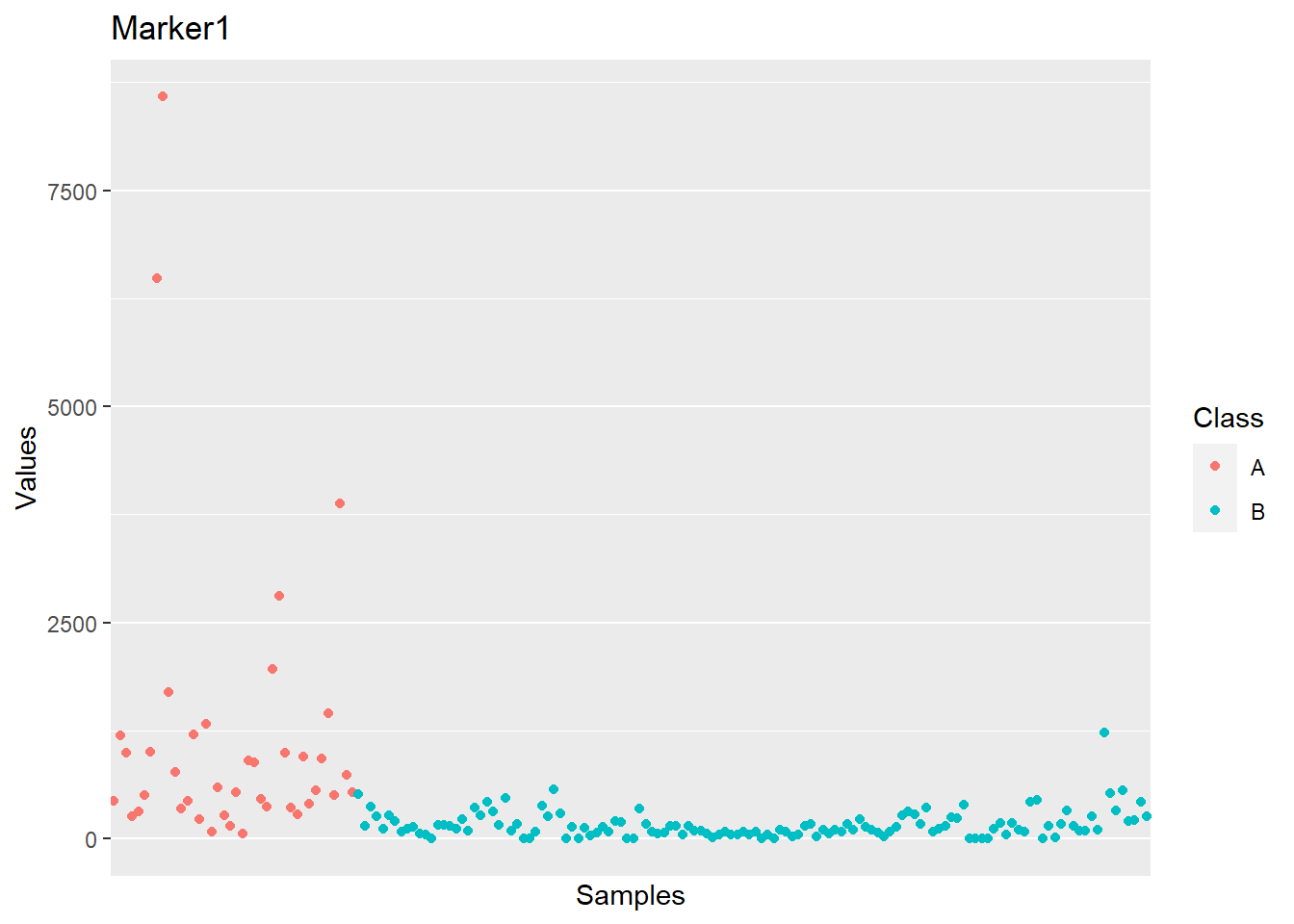In the section “Code snippets” at the end of this vignette we suggest code snippets that can be used to customize the plots for individual markers across all samples, as well as to modify the summary statistics.

Markers distribution overview

Since the target of the analysis is the identification of marker combinations capable to correctly classify samples, the user should first choose a signal threshold to define the positivity for a given marker/combination. This threshold should:

• Positively select most samples belonging to the case class (labelled with “A” in the “Class” column of the dataset), which values must be above the signal threshold.
• Negatively select most control samples (labelled “B”), which values must be below the signal threshold.

Usually this threshold is suggested by the guidelines of the kit used for the analysis (e.g. mean of buffer signal + n standard deviations). However, it is a good practice to always check the distribution of signal intensity of the dataset. To help the user with this operation, the markers_distribution() function have been implemented generating a set of discoverable objects.

This function takes as input the data in long format ( data_long ), and returns a named list (here assigned to the distr object). Please note that the only required argument of markers_distributions() function is case_class, while other arguments have defaults: specific warnings are triggered with this command remembering the users the default threshold parameters that are in place during the computation.

distr <- markers_distribution(data_long, case_class = 'A',
y_lim = 0.0015, x_lim = 3000,
signalthr_prediction = TRUE,
min_SE = 40, min_SP = 80,
boxplot_lim = 2000)
## Warning in markers_distribution(data_long, case_class = "A", y_lim = 0.0015, :
## In $Coord object you will see only the signal threshold values at which SE>=40 ## and SP>=80 by default. If you want to change this limits, please set min_SE and ## min_SP ## Warning in markers_distribution(data_long, case_class = "A", y_lim = 0.0015, : ## The suggested signal threshold in$Plot_density is the median of the signal
## thresholds at which SE>=min_SE and SP>=min_SP. This is ONLY a suggestion. Please
## check if signal threshold is suggested by your analysis kit guidelines instead,
## and remember to check $Plot_density to better judge our suggested threshold by ## inspecting the 2 distributions. The distr object The distr object contains the following elements: • a Boxplot distribution of all single markers • a ROC curve for all markers • the above curve’s coordinates • a density plot for case and control classes • a summary with a few statistics. Once the markers_distributions() function is run, all the abve elements can be plotted or displayed individually. Let’s see each one of them Boxplot The Boxplot shows the distribution of each marker values for both classes: distr$Boxplot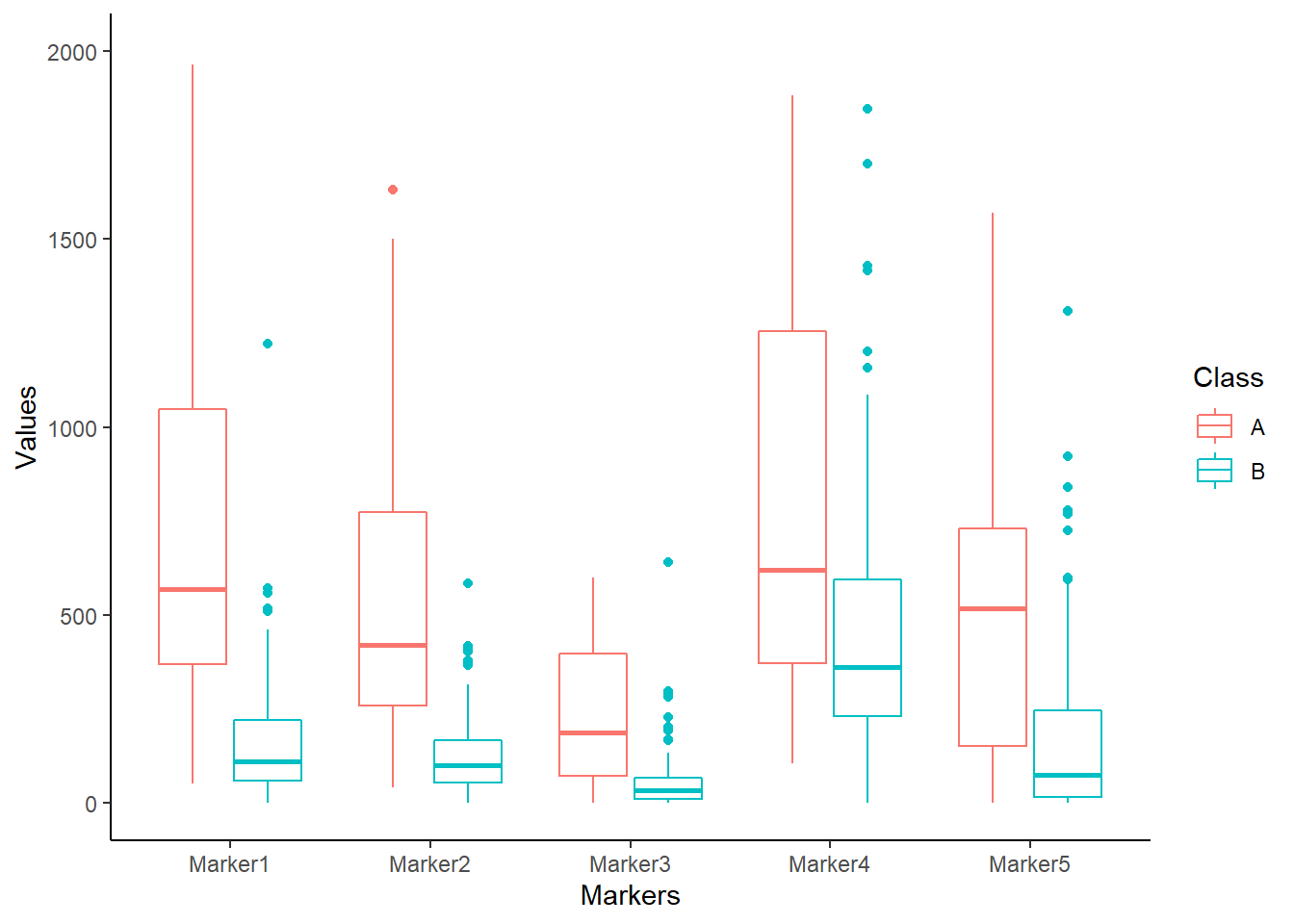The ROC curve for all markers and its coordinates

The ROC curve shows how many real positive samples would be found positive (sensitivity, or SE) and how many real negative samples would be found negative (specificity, or SP) in function of signal threshold. Please note that the False Positive Rate (i.e. 1 - specificity) is plotted on the x-axis. These SE and SP are refereed to the signal intensity threshold considering all the markers together; they are not the SE and SP of a single marker/combination computed by the se_sp() function further discussed in the Sensitivity and specificity paragraph.

distr$ROC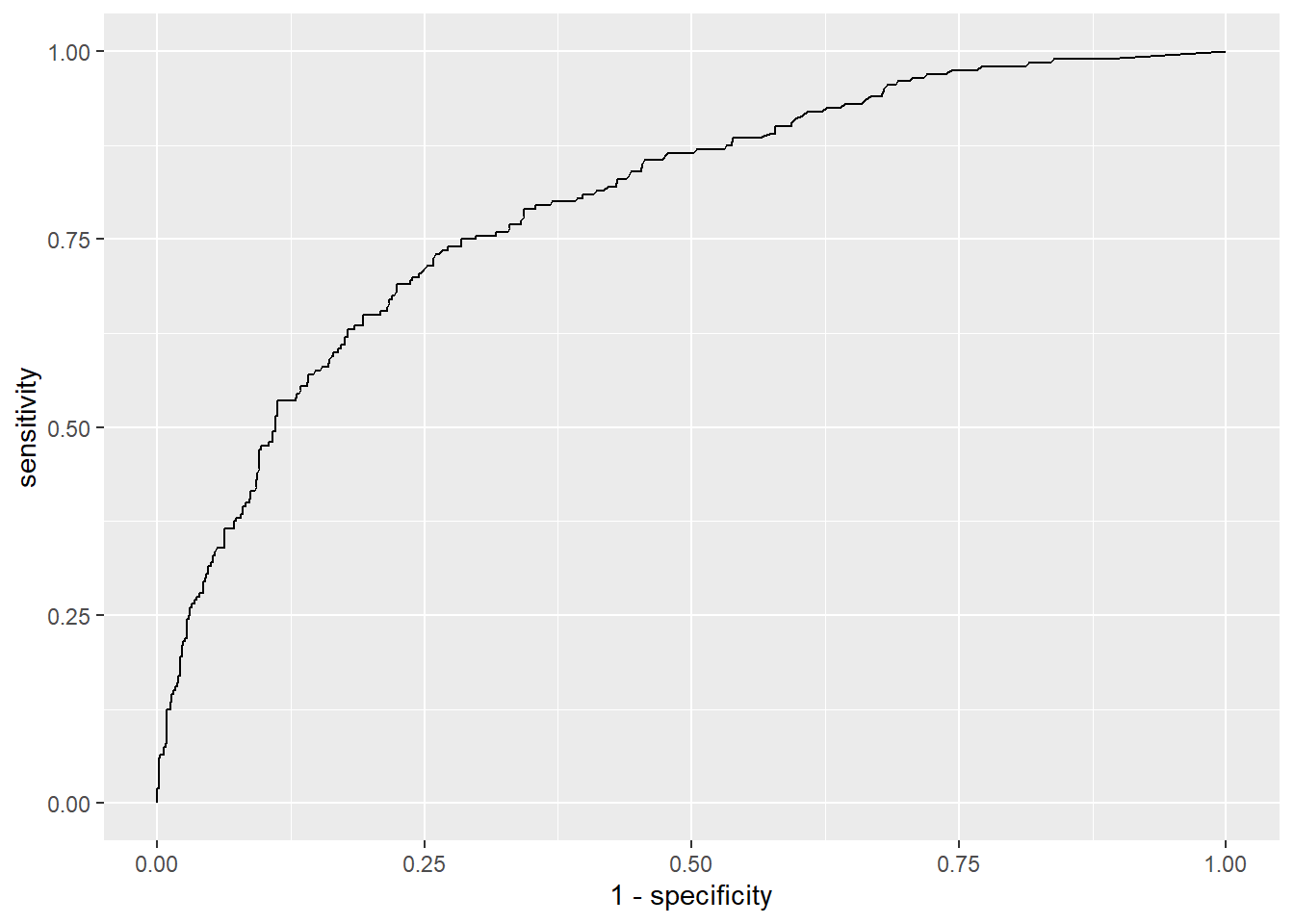The Coord is a dataframe that contains the coordinates of the above described “ROC” (threshold, SP and SE) that have at least a minimun SE (min_SE) and a minimum SP (min_SP): this two threshold are set by default at min_SE = 40 and min_SP = 80, but they can be set manually by specifying different values. The Youden index is also computed: this is the Youden’s J statistic capturing the performance of a dichotomous diagnostic test, with higher values for better performance ( $$J = SE + SP -1$$). head(distr$Coord, n=10)

The density plot and suggested signal threshold

The Density_plot shows the distribution of the signal intensity values for both the classes. In addition, the function allows the user to set both the y_lim and x_lim values to provide a better visualization. One important feature of the density plot is that it calculates a possible signal intensity threshold: in case of lack of a priori knowedge of the threshold the user can set the argument signalthr_prediction = TRUE in the markers_distribution() function. In this way the function calculates a “suggested signal threshold” that corresponds to the median of the signal threshold values (in Coord) at which SE and SP are greater or equal to their set minimal values (min_SE and min_SP). This threshold is added to the “Density_plot” object as a dashed black line and a number. The use of the median allows to pick a threshold whose SE/SP are not too close to the limits (min_SE and min_SP), but it is recommended to always inspect “Coord” and choose the most appropriate signal threshold by considering SP, SE and Youden index.

This suggested signal threshold can be used as signalthr argument of the combi() function further in the workflow.

Combinatorial analysis

combi() function works on the dataset initially loaded. It computes the marker combinations and counts their corresponding positive samples for each class (once thresholds are selected). A sample, to be considered positive for a given combination, must have a value higher than a given signal threshold (signalthr) for at least a given number of markers composing that combination (combithr).

As mentioned before, signalthr should be set depending on the guidelines and characteristics of the methodology used for the analysis or by an accurate inspection of signal intensity distribution. In case of lack of specific guidelines, one should set the value signalthr as suggested by the distr$Density_plot as described in the previous section. In this vignette signalthr is set at 450 while combithr is set at 1. We are setting this at 450 (instead of 407 as suggested by the distr$Density_plot) in order to reproduce the results reported in Mazzara et. al 2017 (the original CombiROC paper) or in Bombaci & Rossi 2019 as well as in the tutorial of the web app with default thresholds.

combithr, instead, should be set exclusively depending on the needed stringency: 1 is the less stringent and most common choice (meaning that at least one marker in a combination needs to reach the threshold). The obtained tab object is a dataframe of all the combinations obtained with the chosen parameters.

tab <- combi(data, signalthr = 450, combithr = 1)

Sensitivity and specificity

Once all the combinations are computed, se_sp() function takes as inputs the loaded data data and the previously obtained set of combinations (tab) to calculate:

• Sensitivity (SE) and specificity (SP) of each combination for each class;
• the number of markers composing each combination (#Markers).

SE of case class (“A”) is calculated dividing the number of positive samples by the total sample of case class (% of positive “A” samples), while case class SP is calculated subtracting SE to 100 (% of negative “A” samples).

SE of control class (“B”) is calculated dividing the number of positive samples by the total sample of control class (% of positive “B” samples), while SP is calculated subtracting SE to 100 (% of negative “B” samples).

Thus, the SE of a given combination (capability to find real positives/cases) corresponds to the SE of the case class (in this case “A”), while its SP (capability to exclude real negatives/controls) corresponds to the SP of the control class (in this case “B”). The obtained value of SE, SP and number of markers are assigned to “markers” mks dataframe.

mks <- se_sp(data, tab)
mks

Selection of combinations

The markers combinations can now be ranked and selected. After specifying the case class (“A” in this case), the function ranked_combs() ranks the combinations by the Youden index in order to show the combinations with the highest SE (of cases) and SP (of controls) on the top, facilitating the user in the selection of the best ones. Again, the Youden index (J) is calculated in this way: $J = SE+SP-1$ The user can also set (not mandatory) a minimal value of SE and/or SP that a combination must have to be selected, i.e. to be considered as “gold” combinations.

A possibility to overview how single markers and all combinations are distributed in the SE - SP ballpark is to plot them with the bubble chart code suggested in the Additional Tips&Tricks section (see: Bubble plot of all combinations) starting from the mks dataframe obtained with the se_sp() function (see above).

The bigger the bubble, the more markers are in the combination: looking at the size and distribution of bubbles across SE and SP values is useful to anticipate how effective will be the combinations in the ranking. Setting no cutoffs (i.e. SE = 0 and SP = 0), all single markers and combinations (all bubbles) will be considered as “gold” combinations and ranked in the next passage.

In the the example below the minimal values of SE and SP are set, respectively, to 40 and 80, in order to reproduce the gold combinations selection reported in Mazzara et. al 2017. The obtained values of combinations, ranked according to Youden index, are stored in the “ranked markers” rmks object containing the table dataframe and the bubble_chart plot that can be accessed individually with the $operator. rmks <- ranked_combs(data, mks, case_class = 'A', min_SE = 40, min_SP = 80) rmks$table

as mentioned, the rmks object also has a slot for the bubble_chart plot, that can be recalled with the usual $operator. This plot discriminates between combinations not passing the SE and SP cutoffs as set in ranked_combs() (blue bubbles) and “gold” combinations passing them (yellow bubbles). rmks$bubble_chart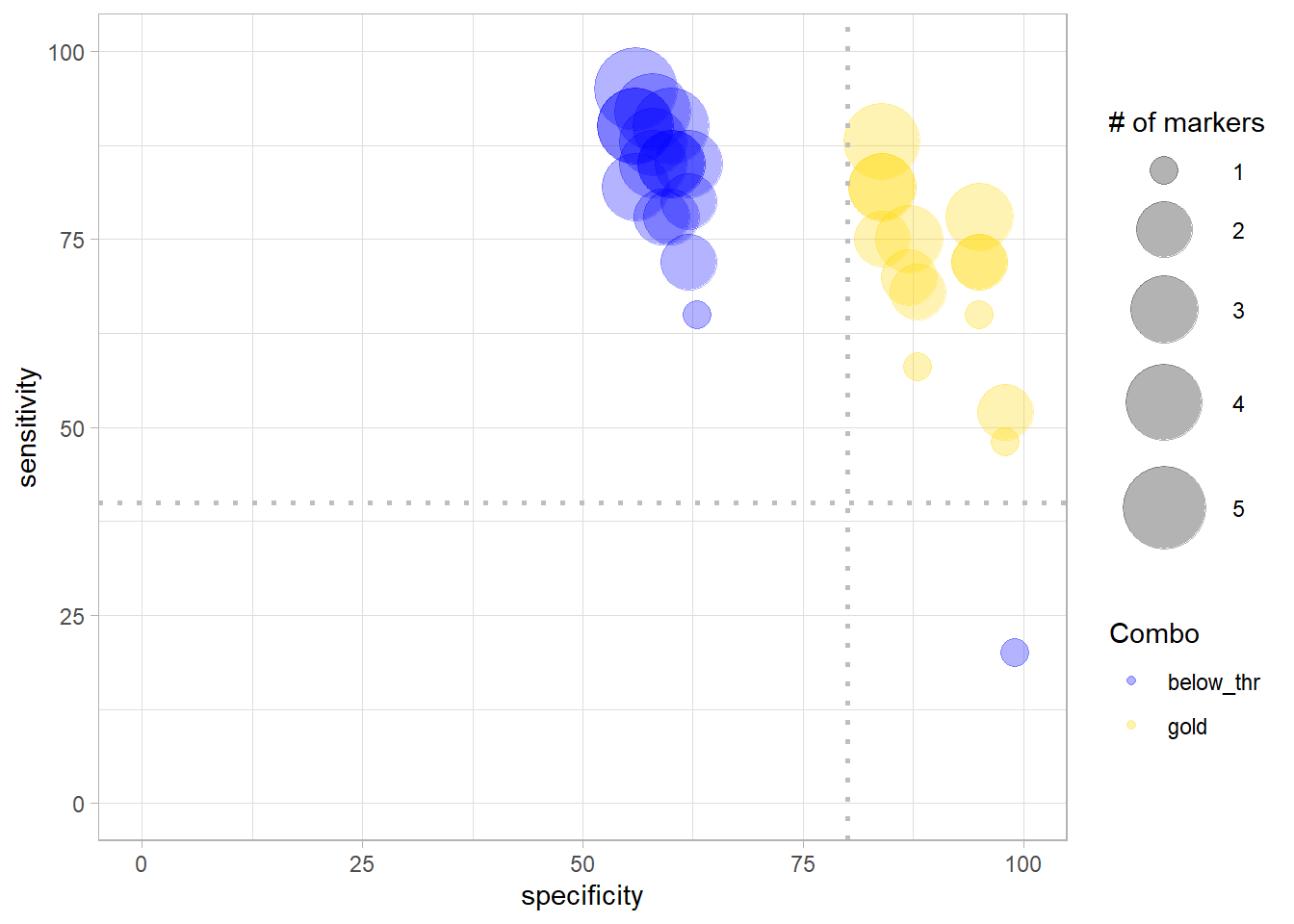ROC curves

To allow an objective comparison of combinations, the function roc_reports() applies the Generalised Linear Model (stats::glm() with argument family= binomial) for each gold combination. The resulting predictions are then used to compute ROC curves (with function pROC::roc()) and their corresponding metrics which are both returned by the function as a named list object (in this case called reports). The function roc_reports() requires as input:

• The data object ( data ) obtained with load_data();
• the table with combinations and corresponding positive samples counts ( tab ), obtained with combi().

In addition, the user has to specify the class case, and the single markers and/or the combinations that she/he wants to be displayed with the specific function’s arguments.
In the example below a single marker ( Marker1 ) and two combinations (combinations number 11 and 15 ) were choosen.

reports <-roc_reports(data, markers_table = tab,
case_class = 'A',
single_markers =c('Marker1'),
selected_combinations = c(11,15))

The obtained reports object contains 3 items that can be accessed using the $operator: • Plot: a ggplot object with the ROC curves of the selected combinations; • Metrics: a dataframe with the metrics of the roc curves (AUC, opt. cutoff, etc …); • Models: The list of models that have been computed and then used to classify the samples (the equation for each selected combination). reports$Plot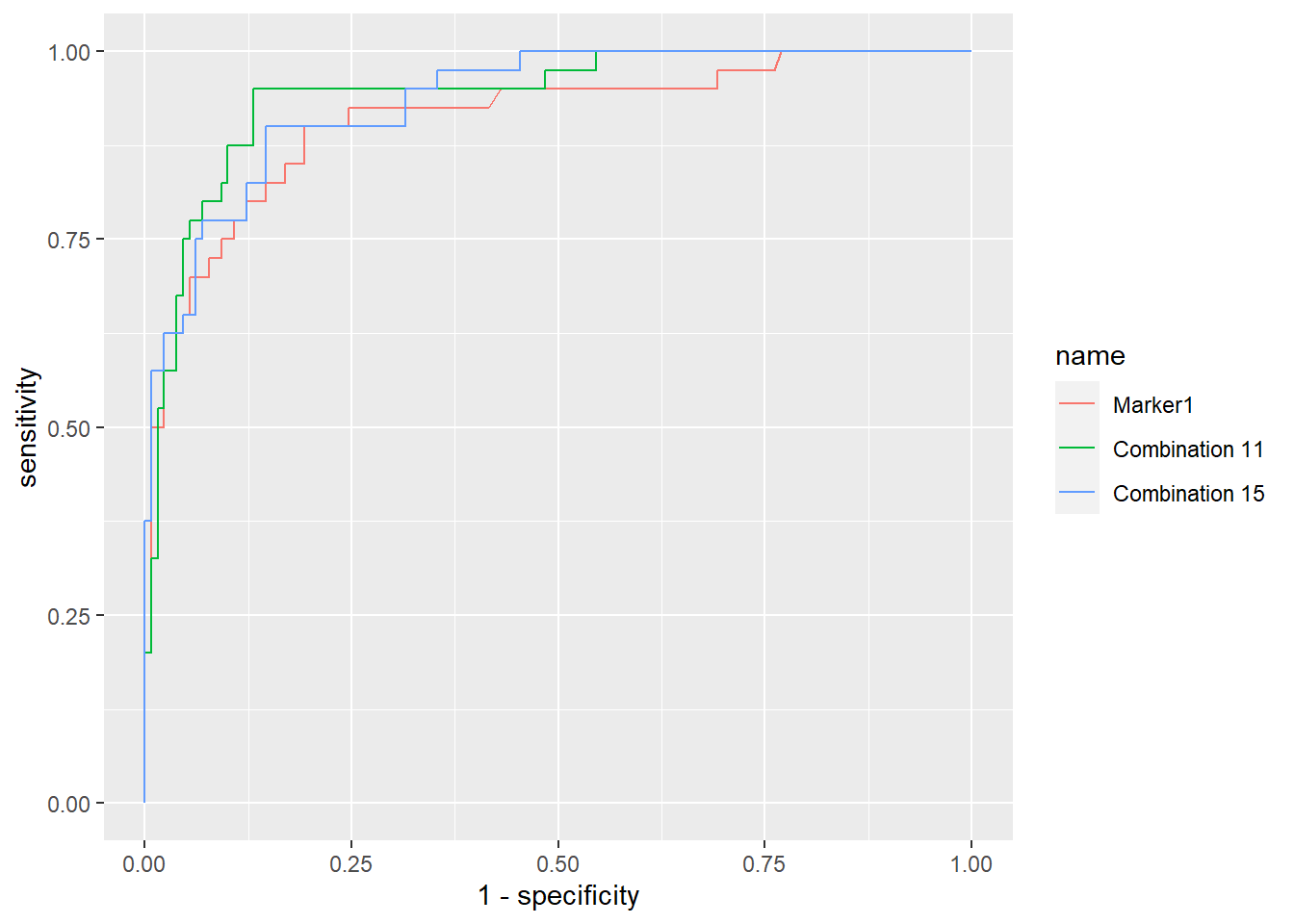reports$Metrics reports$Models
## $Marker1 ## ## Call: glm(formula = fla, family = "binomial", data = data) ## ## Coefficients: ## (Intercept) log(Marker1 + 1) ## -13.775 2.246 ## ## Degrees of Freedom: 169 Total (i.e. Null); 168 Residual ## Null Deviance: 185.5 ## Residual Deviance: 101.7 AIC: 105.7 ## ##$Combination 11
##
## Call:  glm(formula = fla, family = "binomial", data = data)
##
## Coefficients:
##      (Intercept)  log(Marker1 + 1)  log(Marker2 + 1)  log(Marker3 + 1)
##         -17.0128            1.5378            0.9176            0.5706
##
## Degrees of Freedom: 169 Total (i.e. Null);  166 Residual
## Null Deviance:       185.5
## Residual Deviance: 87.49     AIC: 95.49
##
## $Combination 15 ## ## Call: glm(formula = fla, family = "binomial", data = data) ## ## Coefficients: ## (Intercept) log(Marker1 + 1) log(Marker3 + 1) log(Marker5 + 1) ## -16.0554 1.9595 0.6032 0.2805 ## ## Degrees of Freedom: 169 Total (i.e. Null); 166 Residual ## Null Deviance: 185.5 ## Residual Deviance: 87.95 AIC: 95.95 Under the hood For a bit deeper discussion on how to interpret the results, this section will be focused on a single specific combination in the dataset seen so far: “Combination 11”, combining Marker1, Marker2 and Marker3. This combination has an optimal cutoff equal to 0.216 (see the CutOff column in reports$Metrics).
The following is the regression equation being used by the Generalized Linear Model (glm) function to compute the predictions:

$f(x)=β_0+β_1x_1+β_2x_2+ β_3x_3 +...+β_nx_n$

Where $$β_n$$ are the coefficients (being $$β_0$$ the intercept) determined by the model and $$x_n$$ the variables.
While, the predicted probabilities have been calculated with the sigmoid function:

$p(x) = \frac{\mathrm{1} }{\mathrm{1} + e^{-f(x)} }$

In accordance with the above, the predictions for “Combination 11” have been computed using the coefficients displayed as in reports$Models (see previous paragraph), and this combination’s prediction equation will be: $f(x)= -17.0128 + 1.5378 *log(Marker1 + 1) + 0.9176 *log(Marker2 + 1) + 0.5706* log(Marker3 + 1)$ As for the predict method for a Generalized Linear Model, predictions are produced on the scale of the additive predictors. Predictions ($$f(x)$$ values) of Combination 11 can be visualized using the commmand glm::predict with argument type = "link": head(predict(reports$Models$Combination 11, type='link')) # link = f(x) ## 1 2 3 4 5 6 ## 0.166224681 -0.008125528 2.192603482 1.077910194 3.816098810 0.593971602 Prediction probabilities ($$p(x)$$ values, i.e. predictions on the scale of the response) of Combination 11 can be instead visualized using argument type = "response": head(predict(reports$Models$Combination 11, type='response')) # response = p(x) ## 1 2 3 4 5 6 ## 0.5414607 0.4979686 0.8995833 0.7460983 0.9784606 0.6442759 Finally, the comparison between the prediction probability and the optimal cutoff (here 0.216, see the CutOff column for Classification 11 in reports$Metrics) determines the classification of each sample by following this rule:

$C(x) = \begin{cases} 1 & {p}(x) > opt. cutoff \\ 0 & {p}(x) \leq opt.cutoff \end{cases}$

Specifically, for “Combination 11”:

• Samples with $$p(x)$$ higher than 0.216 are classified as “positives” (1).
• Samples with $$p(x)$$ lower or equal to 0.216 are classified as “negatives” (0).

Thus, using 0.216 as cutoff, Combination 11 is able to classify the samples in the dataset with a SE equal to 95.0%, SP equal to 86.9%, and accuracy equal to 88.8% (see ROC curves, reports$Metrics). Classification of new samples A new feature of the CombiROC package (not present in the CombiROC tool Shiny app), offers the possibility to exploit the models obtained with roc_reports() for each selected marker/combination (and assigned to reports$Models) to directly classify new samples that are not labelled, i.e. not assigned to any case or control classes.

The unclassified data set must be similar to the data set used for the previous combinatorial analysis ( i.e. of the same nature and with the same markers, but obviously without the ‘Class’ column).

To load datasets with unclassified samples a specific load_unclassified_data() function was implemented. This function is analogue to load_data() since it loads the same kind of files and it performs the same format checks, with the exception of the Class column which is not present in an unclassified datasets and thus not required.

For purely demonstrative purposes, in the following example a “synthetic” unclassified data set (‘data/unclassified_proteomic_data.csv’) was used: it was obtained by randomly picking 20 samples from the already classified data set (the data). The loaded unclassified sample is here assigned to the unc_data object.
Please note that this unclassified data set lacks the “Class” column but has a Patient.ID column which actually allows the identification of the class but sample names here are not used in the workflow and have labeling purposes to check the prediction outcomes (a “no” prefix identifies healthy/normal subjects while the absence of the prefix identifies affected/abnormal subjects).

unc_data <- load_unclassified_data(data = './data/demo_unclassified_data.csv', sep = ',')

This very same dataset has been included in CombiROC package as an unclassified demo dataset, which can be directly called typing demo_unclassified_data.

The prediction of the class can be achieved with classify(): this function applies the models previously calculated on a classified data set working as training dataset, to the unclassified dataset and classifies the samples accordingly to the prediction probability and optimal cutoff as shown in the Under the hood section.

This classify() function takes as inputs:

• the unclassified data set containing the new samples to be classified (unc_data);
• the list of models reports$Models that have been previously computed by roc_reports() (reports$Models);
• the list of metrics that have been previously computed by roc_reports() (reports$Metrics). In addition the user can set the labels of the predicted class (setting Positive_class and Negative_class), otherwise they will be 1 for positive samples and 0 for the negative samples by default (see the rule shown in the end of the Results explanation section). Here we are setting Positive_class = "affected" and Negative_class = "healthy" The function returns a data.frame (cl_data in the example below), whose columns contain the predicted class for each sample according to the models used (originally in reports$Models); here we are still usign Marker1, Combination 11 and Combination 15.

unc_data <- demo_unclassified_data
cl_data <- classify(unc_data,
Models =  reports$Models, Metrics = reports$Metrics,
Positive_class = "abnormal",
Negative_class = "normal")

As can be observed comparing the outcome in the dataframe with the tag on samples’ names, the single marker Marker1 is not 100% efficient in correctly predicting the class (see mismatch in second row, where the normal sample “no AIH126” is classified as abnormal by Marker1); instead, both Combination 11 and 15 correctly assign it to the right class.

cl_data

Thus, each column of the prediction dataframe contains the prediction outcome of a given model and, along with the samples names (in the index column), can be accessed with the $operator as usual: cl_data$index
##   "AIH33"     "no AIH126" "AIH12"     "no AIH112" "no AIH41"  "no AIH38"
##   "AIH4"      "no AIH32"  "AIH20"     "no AIH13"  "no AIH11"  "no AIH114"
##  "no AIH121" "AIH17"     "AIH14"     "no AIH106" "no AIH67"  "AIH9"
##  "no AIH74"  "AIH16"
cl_data$Combination 11 ##  "abnormal" "normal" "abnormal" "normal" "normal" "normal" ##  "abnormal" "normal" "abnormal" "normal" "normal" "normal" ##  "normal" "abnormal" "abnormal" "normal" "normal" "abnormal" ##  "normal" "abnormal" Ancillary functions Retrieving composition of combinations show_markers() returns a data frame containing the composition of each combination of interest. It requires as input one or more combinations (only their numbers), and the table with combinations and corresponding positive samples counts (“tab”, obtained with combi()). show_markers(selected_combinations =c(11,15), markers_table = tab) Retrieving combinations containing markers of interest combs_with() returns the combinations containing all the markers of interest. It requires as input one or more single marker, and the table with combinations and corresponding positive samples counts (“tab”, obtained with combi()). The list with the combinations containing all the markers is assigned to “combs_list” object. combs_list <- combs_with(markers=c('Marker1', 'Marker3'), markers_table = tab) ## The combinations in which you can find ALL the selected markers have been computed combs_list ##  2 11 14 15 21 22 24 26 Code snippets In this section we offer code insights taking advantage of the long format of the data_long object; starting from here the user can further customize plots and data summaries returned by the combiroc functions. Plotting of intensities of individual markers A quick plotting overview of individual marker values can be done with a scatter plot using the library ggplot2. Here is an example for the marker “Marker2”: library(dplyr) # needed for the filter() function and %>% operator library(ggplot2) myMarker <- "Marker2" data_long %>% filter(Markers == myMarker) %>% ggplot(aes(x= Patient.ID, y=Values)) + geom_point(aes(color=Class)) + labs(title=myMarker, x ="Samples") + scale_x_discrete(labels = NULL, breaks = NULL)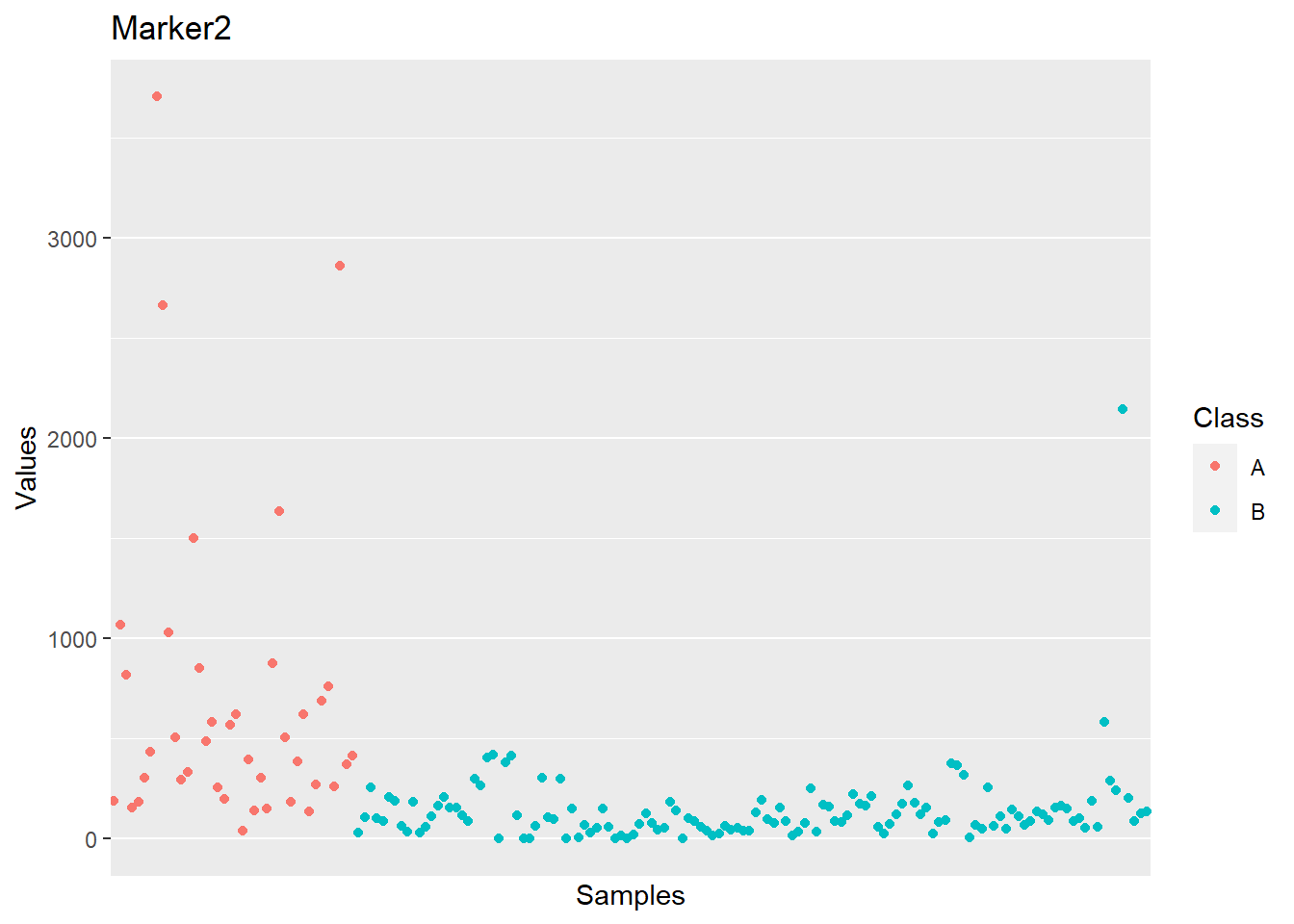Summary statistics of individual markers Further markers exploration can be done using other packages’ functions such as group_by(), summarize() and ggplot() on the the data_long dataframe. As an example, a few summary statistics can be computed for each marker of both classes, separately, as follow (the libraries dplyr and moments are needed). Numbers in this table are those behind the box plot obtained with distr$boxplot as seen before.

library(dplyr)
library(moments) # needed for skewness() function

data_long %>%
group_by(Markers, Class) %>%
summarize(Mean = mean(Values),